# Graphing calculator free for Android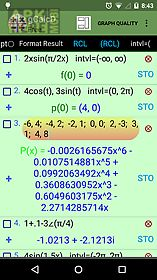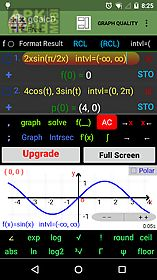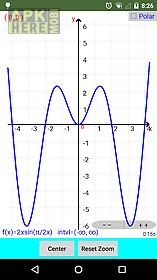Graphing calculator free app for android description: This truly integrated scientific graphing calculator features graphing capabilities offered by no other graphing calculators andits advanced user friendly interface makes graphing and calculations as intuitive as possible.
- Graphing featuresin addition to functions this graphing calculator iscapable of graphing parametric equations and point sets using cartesian and polar coordinate systems.
It is the only free graphing calculator capable offinding and graphing the polynomial of the least degree passing through a set of points.
You can also use this graphing calculator to find and graph the best line that fits a set of points according to gauss 39 least squares criteria.
- Scientific featuresthis calculator is also capable of calculating complex numbers in any form.
The calculator can display a table of values for functions and parametric equations even with complex expressions.
The calculator can display the resultin scientific engineering and fixed point notations.
Calculate relevant expressions in degrees or radians mode.
Apart from standard functions this calculator is equipped with gamma and psi functions.
The calculator also comes with an easy to use units converter (time mass length velocity and many more) and list of constants from various fields of sciences which can be used in your calculations- available functions basic algebraic operations power functions x r and e(x) trigonometric functions and their inverses hyperbolic functions and their inverses exponential e x and exp(x) and logarithmic functions factorial the gamma function and psi function absolutel value and step functions round floor ceil

## Related Apps to Graphing calculator free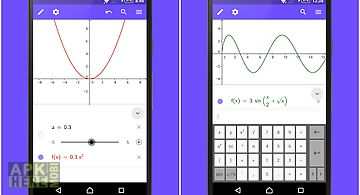Geogebra graphing calculator
Solve math problems graph functions create geometric constructions do statistics and calculus save and share your results. Geogebra apps are used by millio..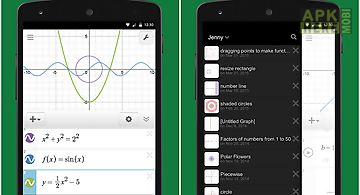Desmos graphing calculator
At desmos we imagine a world of universal math literacy and envision a world where math is accessible and enjoyable for all students. We believe the key is..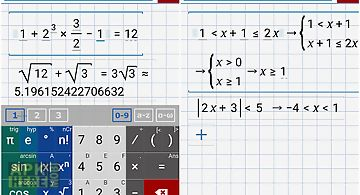Graphing calculator + math
Graphing calculator by mathlab is a scientific graphing calculator integrated with algebra and is an indispensable mathematical tool for students from high..Graphing calculator
I believe every student in the world should have free access to graphing calculator and learn the amazing math and science to the next level. Thanks for yo..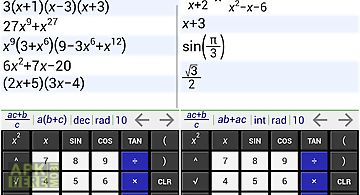Mathally graphing calculator
Mathally graphing calculator is quickly becoming the most comprehensive free graphing symbolic and scientific calculator for android. Here are some of our ..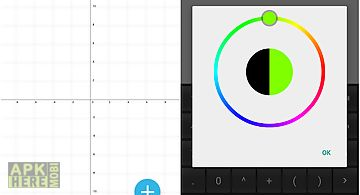Grapher - graphing calculator
Grapher is useful application for all pupils and students. Ease interface will help you to build any graph or function on cartesian coordinate system in fe..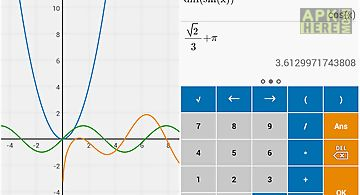Algeo graphing calculator
It has the best graphing experience available on the play store. Draw functions find intersections and show a table of values of the functions with an easy..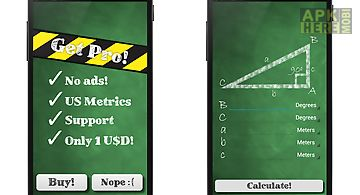Trigonometric calculator
Insert two sides or one angle and one side of a right angled triangle and the trigonometric calculator will do the rest! This is the right angled triangle ..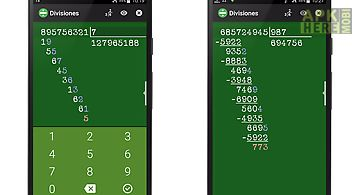Divisions calculator
Simple calculator for divisions for schools showing the remains of each operation. Ideal to learn and fix divisions.Percentages calculator
Simple percentage calculator with big buttons. Ideal for quick calculations. If you like this application you can not miss the full version with more rapid..Statistics calculator
quick easy way to calculate basic statisticsand linear regression import data from csv file easy data entry and data editing with interactive graph quick ..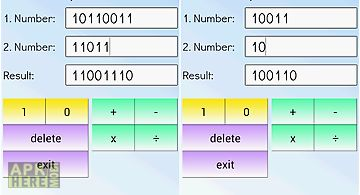Binary calculator
This is a free mathematical calculator which is able to add subtract multiply and divide numbers in binary format (used in computers). Any number can be re..
Apktidy.com - Download free graphing calculator free.apk for Android 4.0.3+ mobile. ApkHere is really the best place to quickly find thousands useful apk apps and new android video games for Samsung Galaxy, Huawei, LG, Sony, Meizu, Asus android phone or tablet devices. All Apk Here apps sort of the most popular categories like education, photo editor, social, fitness, tools apps and brain, card, action, puzzle, online, arcade, racing games in .apk files format. Simple, Fast and safe Apk apps store for your android at Apktidy.com